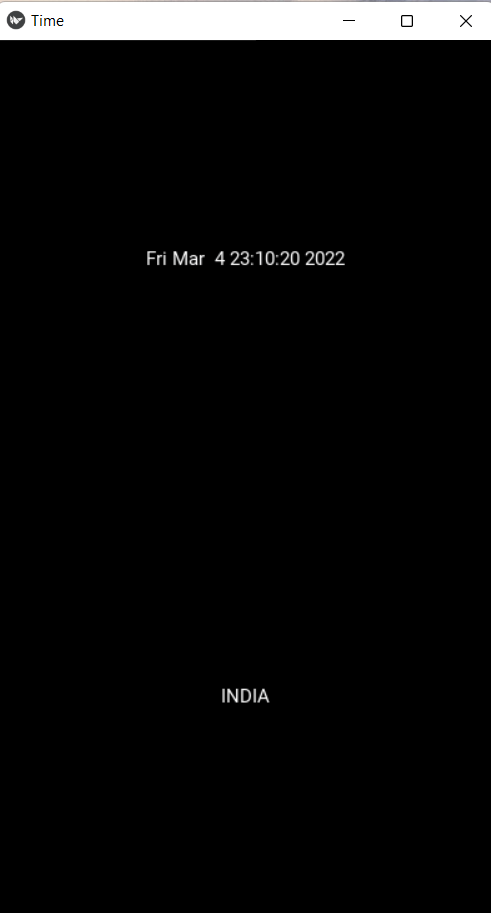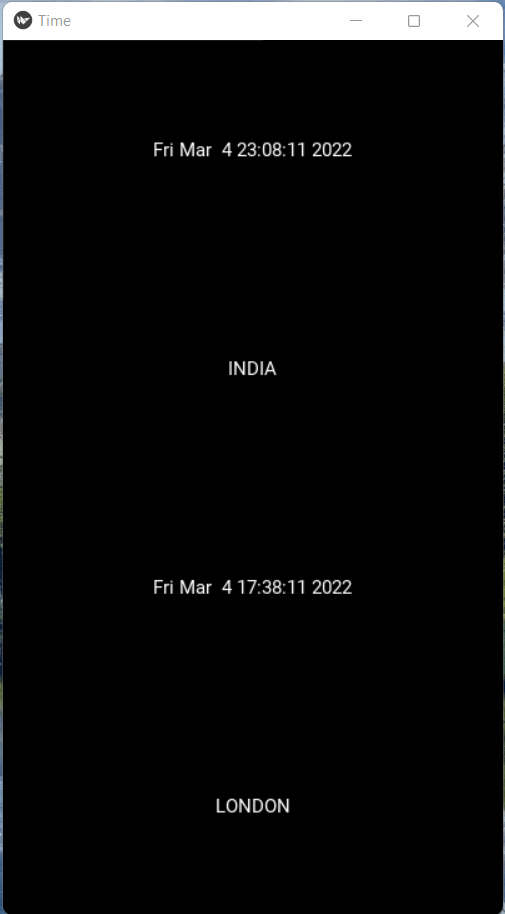GeeksforGeeks App
Open AppBrowser
Continue

# Clock App with Kivy using Python

In this article, we are going to see how to develop a Clock Application with Kivy using Python.

Kivy is a graphical user interface opensource Python library that allows you to develop multi-platform applications on Windows, macOS, Android, iOS, Linux, and Raspberry-Pi. In addition to the regular mouse and keyboard inputs, it also supports multitouch events.

We will be using the time module to get the current time and update it every second. Moreover, we will be also be displaying two times of different timezones.

## Functions Used

• asctime(): By default gets the local time. You can also pass the time zone in it to get the time of another zone as done in Example 2.
• BoxLayout(): It is used to arrange layouts in vertical or horizontal boxes.
• schedule_interval(): Is used to create time intervals and recall the function/event.

## Python3

 `# importing modules` `# it will allow us to get time``import` `time` `# The App class is the base for``# creating Kivy applications``from` `kivy.app ``import` `App` `# it will allow us to make interval calls``from` `kivy.clock ``import` `Clock` `# Label widget will be used to render text``from` `kivymd.uix.label ``import` `Label` `# we will be using this to resize app window``from` `kivy.core.window ``import` `Window` `# it will allow us to create layouts``from` `kivy.uix.boxlayout ``import` `BoxLayout``# declaring window size``Window.size ``=` `(``400``, ``700``)` `# clock class`  `class` `myclock(Label):``    ``def` `update(``self``, ``*``args):` `          ``# get the current local time``        ``self``.text ``=` `time.asctime()` `# App class`  `class` `TimeApp(App):` `    ``def` `build(``self``):``        ``layout ``=` `BoxLayout(orientation``=``'vertical'``)` `        ``# it will create vertical layouts in app` `        ``# calling clock class for time``        ``clock1 ``=` `myclock()` `        ``# updates time with the interval of 1 sec``        ``Clock.schedule_interval(clock1.update, ``1``)` `        ``# adding layout to the screen``        ``layout.add_widget(clock1)` `        ``# adding text to screen``        ``layout.add_widget(Label(text``=``'INDIA'``))` `        ``return` `layout`  `root ``=` `TimeApp()``root.run()  ``# running the app`

Output:Code Explanation

1. The code starts with importing the modules that we need to use.
2. The first module is time which will allow us to get the current local time.
3. Next, it imports the Clock class which will be used for scheduling updates of myclock widget.
4. It then creates a new app called TimeApp and builds it by adding a layout boxlayout and text label.
5. The code is a sample of how to create an application with the Kivy framework.
6. The first line imports modules that will allow us to get time and use it in our code.
7. Line 3 declares the App class which is the base for creating Kivy applications.
8. It allows us to make interval calls, add widgets, and return layouts.
9. Line 4 creates a new instance of myclock widget which renders text.
10. Line 5 schedules 1 second update intervals on clock1 by calling Clock.schedule_interval().
11. Line 6 adds layout to screen with widgets added and returns layout as result of build() function call.
12. Line 7 adds text to screen with Label widget added and returns layout as result of build() function call.

### Example 2: Adding another time zone in the time app

Create another clock class and Add an extra layout for clock 2 in-app class.

## Python3

 `import` `time` `from` `kivy.app ``import` `App``from` `kivy.clock ``import` `Clock``from` `kivymd.uix.label ``import` `Label``from` `kivy.core.window ``import` `Window``from` `kivy.uix.boxlayout ``import` `BoxLayout` `Window.size ``=` `(``400``, ``700``)`  `class` `myclock(Label):``    ``def` `update(``self``, ``*``args):``        ``self``.text ``=` `time.asctime()`  `class` `myclock2(Label):``    ``def` `update(``self``, ``*``args):``        ``t ``=` `time.gmtime()``        ``self``.text ``=` `time.asctime(t)`  `class` `TimeApp(App):` `    ``def` `build(``self``):``        ``layout ``=` `BoxLayout(orientation``=``'vertical'``)` `        ``clock1 ``=` `myclock()``        ``Clock.schedule_interval(clock1.update, ``1``)``        ``layout.add_widget(clock1)``        ``layout.add_widget(Label(text``=``'INDIA'``))` `        ``clock2 ``=` `myclock2()``        ``Clock.schedule_interval(clock2.update, ``1``)``        ``layout.add_widget(clock2)``        ``layout.add_widget(Label(text``=``'LONDON'``))` `        ``return` `layout`  `root ``=` `TimeApp()``root.run()`

Output:Code Explanation:

1. The code starts by creating two Label widgets.
2. The first is myclock1 and the second is myclock2.
3. They are both created with a function called update, which takes no arguments and returns nothing.
4. This means that when they are updated, their text will be set to the current time in asctime().
5. The next step is to create a BoxLayout widget with an orientation of ‘vertical’.
6. It then adds these two Label widgets to it.
7. Finally, it creates another BoxLayout widget but this one has an orientation of ‘horizontal’ and adds those two Label widgets again.
8. The code is designed to create a widget that displays the current time on screen.
9. The first widget, myclock1, will update every second and display the current time in text form.
10. The second widget, myclock2, will update every second and display the current time in text form with an extra line of text at the top displaying the difference between seconds (in this case 1).

My Personal Notes arrow_drop_up